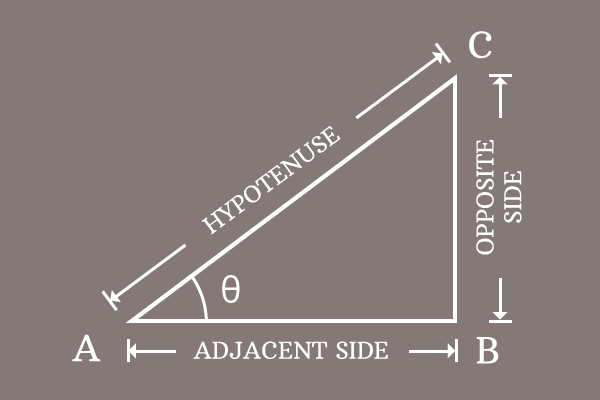# Pythagorean identity of Cosecant and Cotangent functions

## Formula

$\csc^2{\theta}-\cot^2{\theta} \,=\, 1$

The subtraction of square of cot function from square of co-secant function equals to one is called the Pythagorean identity of cosecant and co-tangent functions.

### Introduction

In trigonometry, the cosecant and cotangent are two functions, which have a direct relationship between them in square form but it is derived from Pythagorean theorem. Hence, the relationship between cosecant and cot functions in square form is called the Pythagorean identity of co-secant and co-tangent functions.1. $\Delta BAC$ is a right triangle in which its angle is denoted by the symbol theta.
2. The cosecant and cotangent functions are written as $\csc{\theta}$ and $\cot{\theta}$ respectively.
3. In the same way, their squares are written as $\csc^2{\theta}$ and $\cot^2{\theta}$ respectively in mathematical form.

The subtraction of the cot squared of angle from cosecant squared of angle is equal to one and it is called as the Pythagorean identity of cosecant and cotangent functions.

$\csc^2{\theta}-\cot^2{\theta} \,=\, 1$

#### Popular forms

The Pythagorean identity of cosecant and cot functions is also written popularly in two other forms.

1. $\csc^2{x}-\cot^2{x} \,=\, 1$
2. $\csc^2{A}-\cot^2{A} \,=\, 1$

Remember that the angle of a right triangle can be denoted by any symbol but the relation between cosecant and cot functions have to be written in that symbol.

### Proof

Learn how to prove the Pythagorean identity of cosecant and cot functions in mathematical form by geometrical method.

Latest Math Topics
Jun 26, 2023
Jun 23, 2023

Latest Math Problems
Jul 01, 2023
Jun 25, 2023
###### Math Questions

The math problems with solutions to learn how to solve a problem.

Learn solutions

Practice now

###### Math Videos

The math videos tutorials with visual graphics to learn every concept.

Watch now

###### Subscribe us

Get the latest math updates from the Math Doubts by subscribing us.